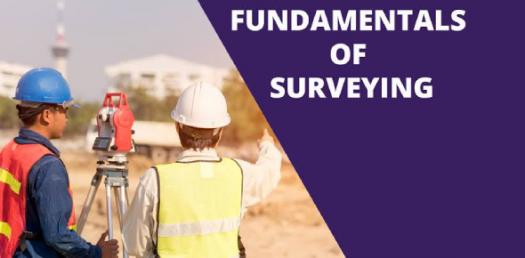# Fundamentals Of Surveying: Test Your Knowledge Trivia Questions Quiz

33 Questions | Attempts: 585
ShareSettingsDo you know the fundamentals of surveying? Do you think that you are so knowledgeable about surveying that you can pass the challenging quiz below on the basis of surveying? The best way to test your knowledge is to take the trivia questions. Do give it a try and get to see what facts you have forgotten already. All the best!

• 1.
Which of the following is a term used in linear surveying?
• A.

Traverse

• B.

Theodolite

• C.

Chainage

• D.

Total Station

• 2.
Which of the following is a term used in levelling surveying?
• A.

Staff

• B.

Traverse

• C.

Offset

• D.

Vertical angle

• 3.
Which of the following is a term used in traverse surveying?
• A.

Staff

• B.

Ranging pole

• C.

Theodolite

• D.

Measuring tape

• 4.
What is a global coordinate system?
• A.

UTM

• B.

ATM

• C.

BM

• D.

CP

• 5.
What is Mean Sea Level (MSL)?
• A.

Average point between high and low tide over time

• B.

Universal Traverse Mercator

• C.

Prime Meridian

• D.

Equator

• 6.
What is a Benchmark (BM)?
• A.

Back sight

• B.

A point of known E,N only

• C.

A point of known elevation

• D.

Inaccessible point

• 7.
What is the height of collimation (HOC) for a Level?
• A.

Back sight

• B.

Height of the instrument after setting up

• C.

A point of known elevation

• D.

• 8.
What is the smallest gradations measurement on a staff in ordinary levelling?
• A.

1.0m

• B.

0.1m

• C.

0.01m

• D.

0.001m

• 9.
What is the first reading that you take after setting up the level?
• A.

FS

• B.

BS

• C.

BM

• D.

IS

• 10.
Where is the best place to set up the level?
• A.

Far away from BS

• B.

Far away from FS

• C.

Where it can target most points if not all

• D.

Near operating heavy machinery

• 11.
What is the correct formula to calculate the reduced level (RL) of last point?
• A.

BS+FS

• B.

IS-FS

• C.

HOC-FS

• D.

HOC+FS

• 12.
What does a contour line represent?
• A.

A group of points with the same elevation

• B.

Horizontal distance between two points

• C.

The angle between two lines

• D.

Levelling grid

• 13.
Which statement is correct for this road cross section?
• A.

Fill only section

• B.

Cut only section

• C.

Cut and fill section

• D.

The formation level is above NGL

• 14.
Which statement is correct for this road cross-section?
• A.

Fill only section

• B.

Cut only section

• C.

Cut and fill section

• D.

The formation level is below NGL

• 15.
Which statement is correct for this road cross-section?
• A.

Fill only section

• B.

Cut only section

• C.

Cut and fill section

• D.

Road does not have a ditch

• 16.
• A.

1.68m

• B.

15m

• C.

16m

• D.

1.58m

• 17.
• A.

1.50m

• B.

15m

• C.

16m

• D.

1.55m

• 18.
• A.

1.64m

• B.

15m

• C.

16m

• D.

1.54m

• 19.
Which line on this contour map has the steepest slope?
• A.

Line A

• B.

Line B

• C.

Line C

• D.

Line D

• 20.
You mark the survey stations using a:
• A.

Staff

• B.

Ranging Pole

• C.

Tripod

• D.

Level

• 21.
You use what type of measurements along the survey line:
• A.

Strolling

• B.

Walking

• C.

Running

• D.

Trotting

• 22.
A perpendicular offset is at?
• A.

30 degrees

• B.

90 degrees

• C.

180 degrees

• D.

270 degree

• 23.
Which of these surveying equipment can be used for linear surveys?
• A.

Staff

• B.

Theodolite

• C.

Measuring tape

• D.

Level

• 24.
Which of these surveying equipment can be used for linear surveys?
• A.

Staff

• B.

Theodolite

• C.

Measuring wheel

• D.

Level

• 25.
It is important to choose an appropriate paper size for drawing surveying maps. What size is A3 paper?
• A.

210mm*297mm

• B.

841mm*1189mm

• C.

594mm*841mm

• D.

297mm*420mm

## Related TopicsBack to top
×

Wait!
Here's an interesting quiz for you.